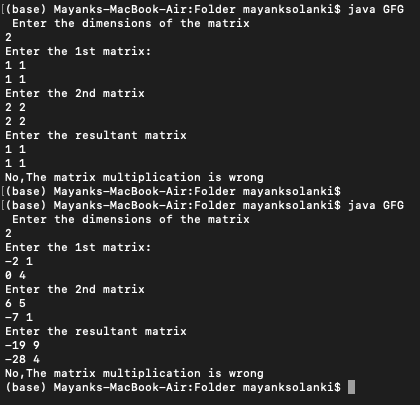Open in App
Not now

# Implementing Coppersmith Freivald’s Algorithm in Java

• Difficulty Level : Expert
• Last Updated : 06 Mar, 2023

Concept: Coppersmith Freivald’s Algorithm is to check whether the matrix A multiplied by matrix B equals the given matrix C. It is used to verify matrix multiplication. It is verified with the help of an equation which stands A*(B*r)-(C*r)=0, where r is a random column vector consisting of 0/1 only.

Illustration:

```Input:

Enter the dimensions of the matrices:

2

Enter the 1st matrix:

-2 1

0  4

Enter the 2nd matrix:

6  5

-7  1

Enter the result matrix:

-19  9

-28  4

Output: Yes, The matrix multiplication is correct.```

### Approach:

Take the size of the matrix as input from the user.

Goal: According to the equation we need to verify matrix A * matrix B = matrix C.

Take inputs of matrix A(n*n) matrix B(n*n) and the resultant matrix C(n*n) as input.

1) Take array r[n] randomly which consists of elements of 0/1 only.

2) Compute matrix B*r, matrix C*r and then matrix A*(matrix B*r) for evaluating the expression matrix A*(matrix B * r) – (matrix C*r)

3) Check if the equation matrix A*(matrix B * r) – (matrix C*r)=0 or not.

4)If it is zero then print “Yes” else print “No”.

Implementation: Input should be taken in the order shown above, else it will lead to wrong results. Below is the example for consideration

## Java

 `// Importing class to create objects``// generating pseudo random numbers``import` `java.util.Random;` `// Importing class to take input from user``import` `java.util.Scanner;` `public` `class` `GFG {``    ``public` `static` `double``[][] multiplyVector(``double``[][] a,``                                            ``double``[][] b,``                                            ``int` `n)``    ``// Method to check the result of the equation.``    ``{``        ``double` `result[][] = ``new` `double``[n][``1``];``        ``for` `(``int` `i = ``0``; i < n; i++) {``            ``for` `(``int` `j = ``0``; j < ``1``; j++) {``                ``for` `(``int` `k = ``0``; k < n; k++) {``                    ``result[i][j]``                        ``= result[i][j] + a[i][k] * b[i][j];``                ``}``            ``}``        ``}``        ``return` `result;``    ``}` `    ``public` `static` `void` `main(String args[])``    ``{``        ``// Driver main method``        ``Scanner input = ``new` `Scanner(System.in);` `        ``System.out.println(``            ``" Enter the dimensions of the matrix"``);``        ``int` `n = input.nextInt();` `        ``// n- size or dimensions of matrix``        ``System.out.println(``"Enter the 1st matrix:"``);` `        ``// Taking input for 1st matrix``        ``double` `a[][] = ``new` `double``[n][n];` `        ``for` `(``int` `i = ``0``; i < n; i++) {``            ``for` `(``int` `j = ``0``; j < n; j++) {``                ``a[i][j] = input.nextDouble();``            ``}``        ``}` `        ``//``        ``System.out.println(``"Enter the 2nd matrix"``);``        ``double` `b[][] = ``new` `double``[n][n];` `        ``// Taking input for second matrix``        ``for` `(``int` `i = ``0``; i < n; i++) {``            ``for` `(``int` `j = ``0``; j < n; j++) {``                ``b[i][j] = input.nextDouble();``            ``}``        ``}` `        ``// Covering up Resultant matrix``        ``System.out.println(``"Enter the resultant matrix"``);``        ``double` `c[][] = ``new` `double``[n][n];``        ``// the resultant matrix``        ``for` `(``int` `i = ``0``; i < n; i++) {``            ``for` `(``int` `j = ``0``; j < n; j++) {``                ``c[i][j] = input.nextDouble();``            ``}``        ``}` `        ``// generating random matrix r consisting of 0/1 only``        ``double``[][] r = ``new` `double``[n][``1``];``        ``Random random = ``new` `Random();``        ``for` `(``int` `i = ``0``; i < n; i++) {``            ``r[i][``0``] = random.nextInt(``2``);``        ``}` `        ``// testing of the standard equation A*(B*r)-(C*r)=0``        ``double` `br[][] = ``new` `double``[n][``1``];``        ``double` `cr[][] = ``new` `double``[n][``1``];``        ``double` `abr[][] = ``new` `double``[n][``1``];``        ``br = multiplyVector(b, r, n);``        ``cr = multiplyVector(c, r, n);``        ``abr = multiplyVector(a, br, n);` `        ``// check for all zeroes in abr``        ``boolean` `flag = ``true``;``        ``// Setting flag with true` `        ``for` `(``int` `i = ``0``; i < n; i++) {``            ``if` `(abr[i][``0``] == ``0``)``                ``continue``;``            ``else` `                ``// Set flag to false(change flag)``                ``flag = ``false``;``        ``}` `        ``// Boolean comparison resulting in message printing``        ``if` `(flag == ``true``)` `            ``System.out.println(``                ``"Yes,The matrix multiplication is correct"``);``        ``else``            ``System.out.println(``                ``"No,The matrix multiplication is wrong"``);``        ``input.close();``    ``}``}`

Output: Custom input for 2 random matrices of order 2Time Complexity : O(kN^2) where N is the size of the matrix.

My Personal Notes arrow_drop_up